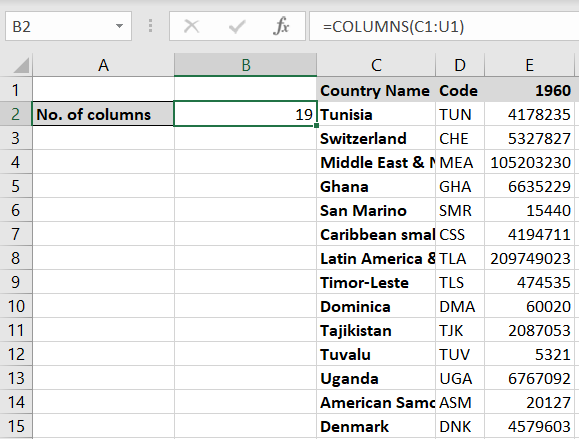# How to count columns in Excel

You can watch a video tutorial here.When working with data in Excel, you may need to count the number of columns either to understand the size of a dataset or to use it in a Formula. To do this, you can use the COLUMNS() function. The syntax is:

COLUMNS(array)

>array: the range of cells for which the columns are to be counted

### Step 1 – Create the formula– Select the cell in which the result is to appear
– Type the formula: